# 实用且奇特的自动交易技术

### 内容目录

• 概述
• 部分持仓平仓算法，利用最后一根柱线的走势
• 混合手数变化算法
• 均摊原理
• 交易技术稳固性的直接和间接迹象
• 利用余额和净值波动
• 结束语

### 部分持仓平仓算法，利用最后一根柱线的走势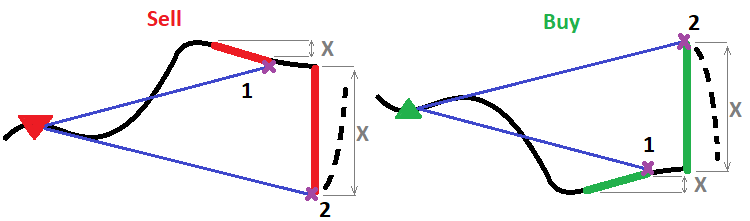• { D } - 幂
• { X } - 前一根柱线走势的点数
• { C } - 比例因子
• { Lc(X) = C * Pow(X , D) } - 一个幂函数，计算在当前烛条处需平仓的手数
• { Lc(X) = C * X } - 一个线性函数，计算在当前烛条处需平仓的手数

• { StartLotsToOnePoint } - 当 X = 1 (输入参数) 时，依据该值平仓
• { PointsForEndLots } - 前一根烛条走势指向盈利方向，则最后持仓平仓速度（输入参数）
• { EndLotsToOnePoint } - 当 X = PointsForEndLots （输入参数）时，依据该值平仓

1. { Lc( ) = StartLotsToOnePoint }
2. { Lc( PointsForEndLots ) = EndLotsToOnePoint }

1. { C * Pow(1 , D) = StartLotsToOnePoint }
2. { C * Pow( PointsForEndLots  , D) = EndLotsToOnePoint }

• { CStartLotsToOnePoint }

• { log( PointsForEndLots ) [ Pow( PointsForEndLots  , D)] = log( PointsForEndLots ) [  EndLotsToOnePoint C ] }

• { D =  log( PointsForEndLots ) [  EndLotsToOnePoint C ] }

• { D = ln(  EndLotsToOnePoint C ) ln(  PointsForEndLots  ) }

• { D = ln(  EndLotsToOnePoint StartLotsToOnePoint  )  ln(  PointsForEndLots  ) }

• { Lc(X) = StartLotsToOnePoint  * Pow( X ,  ln(  EndLotsToOnePoint StartLotsToOnePoint  )  ln(  PointsForEndLots  )  )  }

```double CalcCloseLots(double orderlots0,double X)
{
double functionvalue;
double correctedlots;
if ( X < 0.0 ) return 0.0;
functionvalue=StartLotsToOnePoint*MathPow(X ,MathLog(EndLotsToOnePoint/StartLotsToOnePoint)/MathLog(PointsForEndLots));
correctedlots=GetLotAniError(functionvalue);
if ( correctedlots > orderlots0 ) return orderlots0;
else return correctedlots;
}
```

```void PartialCloseType()// close order partially
{
bool ord;
double ValidLot;
MqlTick TickS;
SymbolInfoTick(_Symbol,TickS);

for ( int i=0; i<OrdersTotal(); i++ )
{

if ( ord && OrderMagicNumber() == MagicF && OrderSymbol() == _Symbol )
{
if ( OrderType() == OP_BUY )
{
ValidLot=CalcCloseLots(OrderLots(),(Open-Open)/_Point);
if ( ValidLot > 0.0 ) ord=OrderClose(OrderTicket(),ValidLot,TickS.bid,MathAbs(SlippageMaxClose),Green);
}
if ( OrderType() == OP_SELL )
{
ValidLot=CalcCloseLots(OrderLots(),(Open-Open)/_Point);
if ( ValidLot > 0.0 ) ord=OrderClose(OrderTicket(),ValidLot,TickS.ask,MathAbs(SlippageMaxClose),Red);
}
break;
}
}
```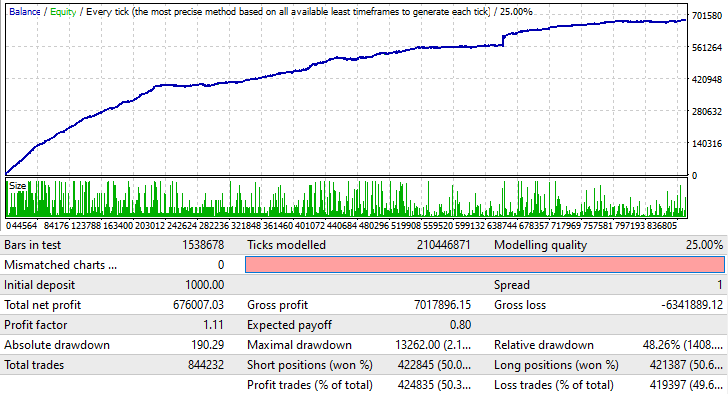### 混合手数变化算法

• 马丁格尔
• 逆马丁格尔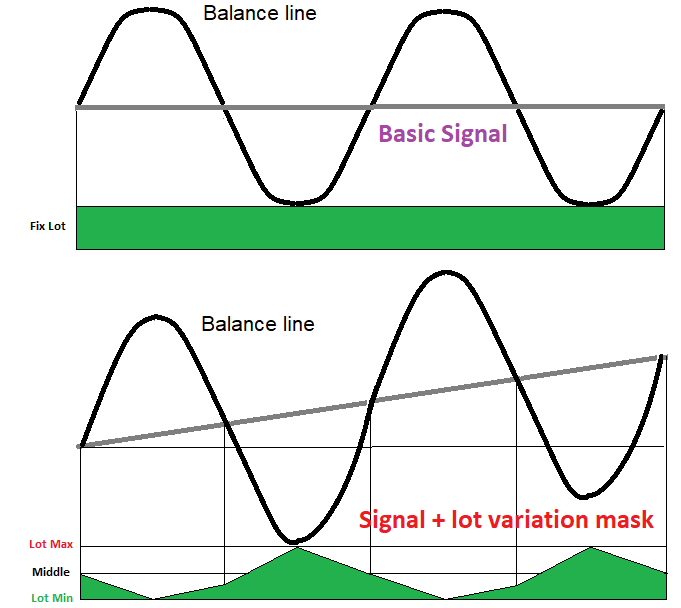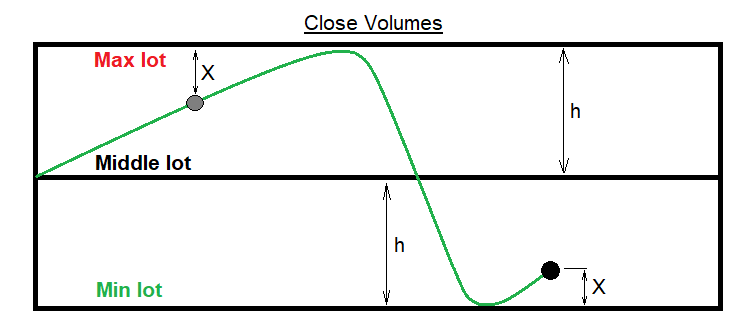• { MaxLot } - 最大手数走廊（输入参数）
• { MinLot } - 最小手数走廊（输入参数）
• { LotForMultiplier } - 增加或减少交易量的参考手数
• { ProfitForMultiplier } - 亏损和获利点数，据其增加或减少参考手数

• MiddleLot = (MaxLot + MinLot)/2 } - 最高和最低手数间的中值
• { h(MaxLot MinLot)/2 } - 通道的一半宽度（用于计算）
• { L } - 历史上最后一笔交易的手数
• { X } - 辅助变量
• { OrderProfit , OrderComission , OrderSwap , OrderLots } - 排除了佣金的利润、佣金、计算出的订单隔夜利息、和历史记录中最后一笔订单平仓量（它们均已知）
• { TickSize } - 假设持仓量等于 1 手，且价格朝理想方向移动了 1 个点，持仓递增的利润
• { PointsProfit( OrderProfit + OrderComission + OrderSwap ) / OrderLots * TickSize ) } - 历史上最后一笔订单的利润，转换为点数

• { L  >=  MiddleLot  ?  X = MaxLot - L  } - 如果手数处于通道的上部，则 “X” 值是与通道上边界的差值
• L  <  MiddleLot  ?  X = L - MinLot } - 如果手数处于通道的下部，则 “X” 值是与通道下边界的距离
• { L  >=  MiddleLot & PointsProfit < 0   ?  Lo(X)OrderLots - LotForMultiplier * (  PointsProfit / ProfitForMultiplier ) * X / h )  } - 接近通道的上边界时，慢速手数增加
• L  <  MiddleLot & PointsProfit >= 0   ?  Lo(X) =  OrderLots - LotForMultiplier * (  PointsProfit / ProfitForMultiplier ) * X / h )  } - 接近通道下边界时，慢速手数减少
• L  >=  MiddleLot & PointsProfit >= 0   ?  Lo(X) =  OrderLots - LotForMultiplier * (  PointsProfit / ProfitForMultiplier ) / X / h )  )  } - 加速手数减少
• L  <  MiddleLot & PointsProfit < 0   ?  Lo(X) =  OrderLots - LotForMultiplier * (  PointsProfit / ProfitForMultiplier ) / X / h )  } - 加速手数增加

```double CalcMultiplierLot()
{
bool ord;
double templot=(MaxLot+MinLot)/2.0;
for ( int i=OrdersHistoryTotal()-1; i>=0; i-- )
{
ord=OrderSelect( i, SELECT_BY_POS, MODE_HISTORY );
if ( ord && OrderSymbol() == CurrentSymbol &&  OrderMagicNumber() == MagicF )
{
double PointsProfit=(OrderProfit()+OrderCommission()+OrderSwap())/(OrderLots()*MarketInfo(CurrentSymbol,MODE_TICKVALUE));
if ( OrderLots() >= (MaxLot+MinLot)/2.0 )
{
if ( PointsProfit < 0.0 ) templot=OrderLots()-(LotForMultiplier*(PointsProfit/ProfitForMultiplier))*((MaxLot-OrderLots())/((MaxLot-MinLot)/2.0));
if ( PointsProfit > 0.0 ) templot=OrderLots()-(LotForMultiplier*(PointsProfit/ProfitForMultiplier))/((MaxLot-OrderLots())/((MaxLot-MinLot)/2.0)) ;
if ( PointsProfit == 0.0 ) templot=OrderLots();
break;
}
else
{
if ( PointsProfit > 0.0 ) templot=OrderLots()-(LotForMultiplier*(PointsProfit/ProfitForMultiplier))*((OrderLots()-MinLot)/((MaxLot-MinLot)/2.0));
if ( PointsProfit < 0.0 ) templot=OrderLots()-(LotForMultiplier*(PointsProfit/ProfitForMultiplier))/((Orderlots()-MinLot)/((MaxLot-MinLot)/2.0));
if ( PointsProfit == 0.0 ) templot=OrderLots();
break;
}
}
}
if ( templot <= MinLot ) templot=(MaxLot+MinLot)/2.0;
if ( templot >= MaxLot ) templot=(MaxLot+MinLot)/2.0;

return templot;
}
```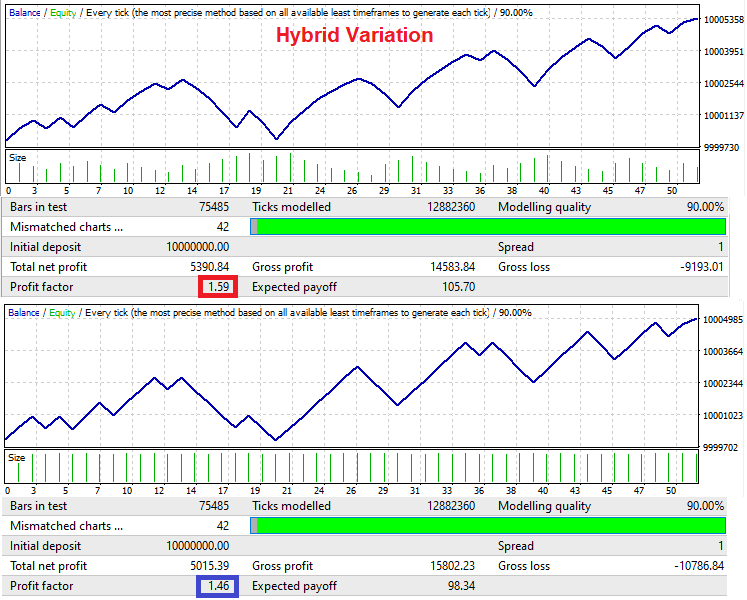### 均摊原理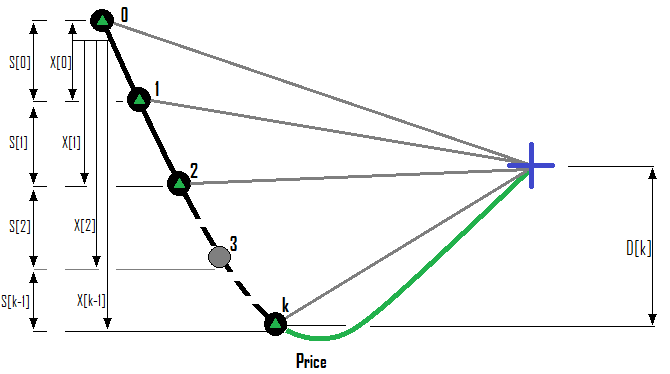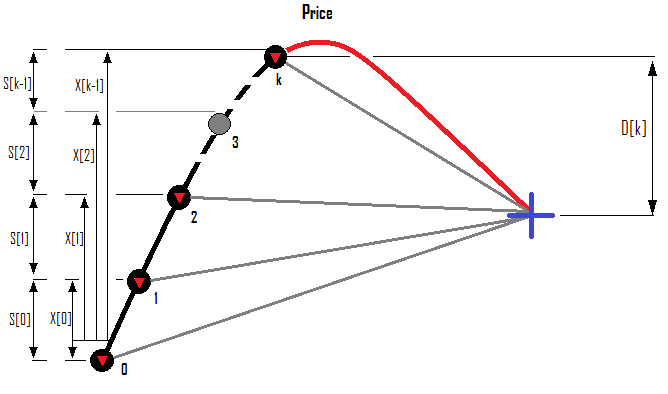• { MaxOrders } - 系列中允许的最大订单数
• {  k = 0 ... 1 ... 2 ...  (MaxOrders-1)  } - 系列中下一笔订单的索引，如果我们未能从之前的方式法获利，其为我们打算开立的订单
• {  ProfitFactorMax } - 允许平仓的盈利因子
• {  i = 1 ... 1 ... 2 ... k  } - 已经存在的扇骨形订单，和我们要开立的下一笔订单的索引
• {  S[i-1]  } - 从上一笔订单到当前订单的点数距离
• {  X[i-1]  } - 从系列的零号订单到当前的点数距离
• {  D[k]  } - 相对于系列最后一笔订单的开单价，预测所需方向上的回滚

• {  P[i] } - 在不排除佣金和隔夜利息的情况下，特定订单的利润
• {  С[i] } - 选定订单的佣金
• {  S[i] } - 所计算订单每天零点时的滚动隔夜利息
• L[i] } - 订单交易量
• Sp[i] } - 开买单时的点差/卖单的当前点差
• { TickSize } - 假设持仓量等于 1 手，并且价格朝期望的方向移动了 1 个点，持仓增加的利润

• {  j = 0 ... 1 ... 2 ... k-1 }
• {  PF[j] = P[j] + С[j] + S[j] + (D[k] * L[j] * TickSize) } - 当所需回滚发生时，指定订单的利润将等于该值
• {  PF[k] = { ( D[k] - Spread[k] ) * L[k]TickSize } - 无法计算此值，因为它包含我们要开仓所需的手数；但是稍后需要用到此表达式

• {  i = 0 ... 1 ... 2 ... k } - 已开单的索引
• {  Pr[i] >= 0, Ls[i]  >= 0 } - 引入 2 个数组，根据品种接受订单盈亏（“+” 将用于获利，这是计算盈利因子所必需的）
• {  PF [i] < 0  ?  Pr[i] = 0 & Ls[i] = - PF[i]  } - 如果订单利润为负，则将其利润写为 “0”，而利润值应用一个负号写入亏损变量
•  PF [i] > 0 ?  Ls[i] = 0 & Pr[i]PF[i]  } - 如果订单获利为正，只需将其写入相应的数组，并在亏损数组中设置零值

• { SummProfit = Summ[0,k]( PF[i] ) } - 所有获利订单的总利润模块
• { SummLoss = Summ[0,k]( Ls [i] ) } - 所有亏损订单的总亏损模块

• { SummLoss > 0 ?  SummProfit/SummLoss  = ProfitFactorMax }

• { SummProfit = Summ[0,k-1]( PF[j] )  +  PF[k] }

• {  ( Summ[0,k-1]( PF[j] )  +  PF[k] ) /  SummLoss  =  ProfitFactorMax }

• { PF[k]  =  ProfitFactorMax * SummLoss  -  Summ[0,k-1]( PF[j] ) }

• { ( D[k] - Spread[k] ) * L[k] TickSize = ProfitFactorMax * SummLoss  -  Summ[0,k-1]( PF[j] ) }

• { L[k] =  ( ProfitFactorMax * SummLoss  -  Summ[0,k-1]( PF[j] ) )  /  ( ( D[k] - Spread[k] ) *  TickSize ) }

• { P[i] = ( X[i-1] - Spread[i] ) * L[i] * TickSize + С[j] + S[j] }

• { D=D(X) }
• { D[k] = D( X[k-1] ) }

• { D = K * X }
• { D = DMin + C * Pow(S , X) }

1. { K } - 线性函数的回滚系数（也用作自动系统的输入参数）
2. { DMin } - 允许的最小回滚（对于幂函数，D >= DMin）
3. { C } - 幂函数伸缩因子
4. { S } - 指数的基数

1. { HalfX } - 附加回滚至价格走势的一半变动（控制函数的附加输入参数）
2. { D(0) = DMinK*DMin }
3. { D(HalfX) = DMin K*DMin/2 }

• { D >= DMin }
• { Lim( X -> +infinity ) = DMin }

1. DMin + C * Pow(S , 0) = DMin K*DMin
2. { DMin + C * Pow(S , HalfX) =  DMin K*DMin/2 }

• { С = K * DMin }

• { Pow(S , HalfX) = 0.5 }

• { S = Pow(0.5  , 1/HalfX) }

• { D(X) = DMinK *  DMin * Pow( Pow(0.5  , 1/HalfX)  , X ) }

```double D(double D0,double K0,double H0,double X)
{
return D0+(D0*K0)*MathPow(MathPow(0.5,1.0/H0),X);
}
```

```double CalculateTranslation()
{
bool ord;
bool bStartDirection;
bool bFind;
double ExtremumPrice=0.0;

for ( int i=0; i<OrdersTotal(); i++ )
{
if ( ord && OrderMagicNumber() == MagicF && OrderSymbol() == Symbol() )
{
if ( OrderType() == OP_SELL ) bStartDirection=false;
if ( OrderType() == OP_BUY ) bStartDirection=true;
ExtremumPrice=OrderOpenPrice();
bFind=true;
break;
}
}
for ( int i=0; i<OrdersTotal(); i++ )
{

if ( ord && OrderMagicNumber() == MagicF && OrderSymbol() == Symbol() )
{
if ( OrderType() == OP_SELL && OrderOpenPrice() > ExtremumPrice ) ExtremumPrice=OrderOpenPrice();
if ( OrderType() == OP_BUY && OrderOpenPrice() < ExtremumPrice ) ExtremumPrice=OrderOpenPrice();
}
}
if ( bFind )
{
if ( bStartDirection ) return (ExtremumPrice-Close)/_Point;
else return (Close-ExtremumPrice)/_Point;
}
else return -1.0;
}
```

```double Xx;//shift of X
double Dd;//desired rollback of D
void CalcXD()//calculate current X and D
{
bool ord;
bool bStartDirection=false;
bool bFind=false;
double ExtremumPrice=0.0;

for ( int i=0; i<OrdersTotal(); i++ )
{
if ( ord && OrderMagicNumber() == MagicF && OrderSymbol() == Symbol() )
{
if ( OrderType() == OP_SELL ) bStartDirection=false;
if ( OrderType() == OP_BUY ) bStartDirection=true;
ExtremumPrice=OrderOpenPrice();
bFind=true;
break;
}
}

for ( int i=0; i<OrdersTotal(); i++ )
{

if ( OrderMagicNumber() == MagicF && OrderSymbol() == Symbol() )
{
if ( OrderType() == OP_SELL && OrderOpenPrice() < ExtremumPrice ) ExtremumPrice=OrderOpenPrice();
if ( OrderType() == OP_BUY && OrderOpenPrice() > ExtremumPrice ) ExtremumPrice=OrderOpenPrice();
}
}
Xx=0.0;
Dd=0.0;
if ( bFind )
{
if ( !bStartDirection ) Xx=(Close-ExtremumPrice)/_Point;
if ( bStartDirection ) Xx=(ExtremumPrice-Close)/_Point;
if ( MODEE==MODE_SINGULARITY ) Dd=D(DE,KE,XE,Xx);
else Dd=Xx*KE;
}
}
```

```double TotalProfitPoints=0.0;
double TotalProfit=0;
double TotalLoss=0;
```

```void CalcLP()//calculate losses and profits of all open orders
{
bool ord;
double TempProfit=0.0;
TotalProfit=0.0;
TotalLoss=0.0;
TotalProfitPoints=0.0;

for ( int i=0; i<OrdersTotal(); i++ )
{

if ( ord && OrderMagicNumber() == MagicF && OrderSymbol() == Symbol() )
{
TempProfit=OrderProfit()+OrderCommission()+OrderSwap();
if ( TempProfit >= 0.0 ) TotalProfit+=TempProfit;
else TotalLoss-=TempProfit;
}
}
}

void CalcLPFuture()//calculate losses and profits of all existing orders in the future
{
bool ord;
double TempProfit=0;
TotalProfit=0;
TotalLoss=0;

for ( int i=0; i<OrdersTotal(); i++ )
{

if ( ord && OrderMagicNumber() == MagicF && OrderSymbol() == Symbol() )
{
if ( TempProfit >= 0.0 ) TotalProfit+=TempProfit;
else TotalLoss-=TempProfit;
}
}
}
```

```bool bClose()
{
CalcLP();
if ( TotalLoss != 0.0 && TotalProfit/TotalLoss >= ProfitFactorMin ) return true;
if ( TotalLoss == 0.0 && TotalProfitPoints >= DE*KE ) return true;
return false;
}
```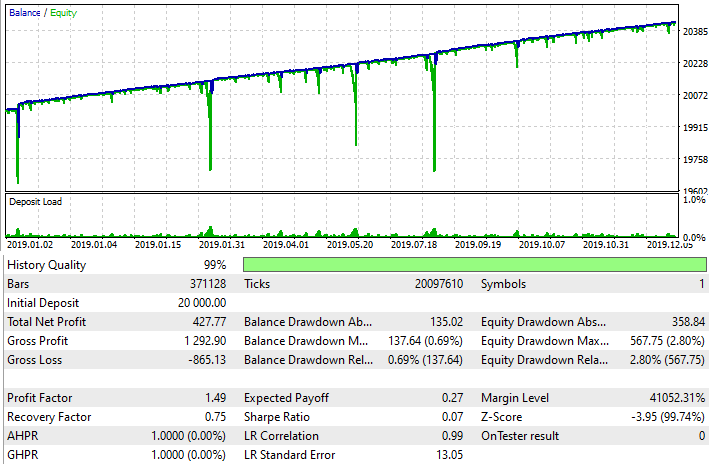### 交易技术稳固性的直接和间接迹象

1. 数据样本的大小，以及回测中的交易数量，在进行统计评估时应最大化
2. 回测值即使略有变化也能表现出正值变化

1. 这种交易技术可运用在任何交易金融产品上
2. 可将多种技术合并起来变成混合技术
3. 如果运用得当，即使存在点差，您盈利的机会也比蒙受损失的机会更大。

• 最终回测利润
• 最大余额回撤
• 最大净值回撤

• 平均余额回撤
• 平均净值回撤

• { i = 0 ... 1 ... 2 ... m } - 半波的次数（半波是一个增长的净值或余额区段，后随下跌区段）
• { j = 0 ... 1 ... 2 ... k } - 负半波区段（余额区段在开仓和平仓点处开始和结束，净值区段在其他点处开始和结束）
• { OrderProfit[j]-OrderComission[j]-OrderSwap[j] < 0 !  Lim( m --> +infinity ) [ Summ(i) [Summ(j) [ OrderProfit[j]-OrderComission[j]-OrderSwap[j] ]] / m  ] = 扣除佣金点差和隔夜利息后的总利润 } - 回测或交易余额的平均回撤
• { SegmentEquity[j]-SegmentComission[j]-SegmentSwap[j] < 0 !  Lim( m --> +infinity ) [ Summ(i) [Summ(j) [ SegmentEquity[j]-SegmentComission[j]-SegmentSwap[j] ]] /  ] = 扣除佣金点差和隔夜利息后的总利润     } - 回测或交易余额的平均回撤

• { Tp } - 最终回测或交易的利润
• { BdM } -  平均余额回撤
• { EdM } - 平均净值回撤
• { BdMax } - 最大余额回撤
• { EdMax } - 最大净值回撤

• { A = Tp/BdM } - 如果该策略不能预测未来，则等于一；如果它知道如何预测并盈利，则等于大于一（相当于回答有关其获利能力的问题）
• { B = Tp/EdM } - 与前值相同
• { C = Tp / BdMax } - 如果在该量具里有所提高，那么我们可以得出结论，该技术提高了该方法的有效性（降低则意味着负面影响）
• { D = Tp / EdMax } - 与前值相同

### 利用余额和净值波动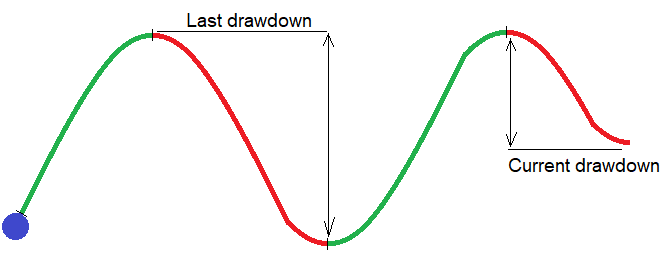• { DealsMinusToBreak } - 前一个周期的亏损交易数量，达到该数量时应将周期的起始手数重置为起始值
• LotDecrease } - 当交易历史中出现新周期时，降低周期起始手数的步长

```double DecreasedLot=Lot;//lot to decrease
double CalcSmartLot()//calculate previous cycle
{
bool ord;
int PrevCycleDeals=0;
for ( int i=HistoryDealsTotal()-1; i>=0; i-- )
{
ulong ticket=HistoryDealGetTicket(i);
ord=HistoryDealSelect(ticket);
if ( ord && HistoryDealGetString(ticket,DEAL_SYMBOL) == _Symbol
&& HistoryDealGetInteger(ticket,DEAL_MAGIC) == MagicC
&& HistoryDealGetInteger(ticket,DEAL_ENTRY) == DEAL_ENTRY_OUT )
{
if ( HistoryDealGetDouble(ticket,DEAL_PROFIT) > 0 )
{
for ( int j=i+1; j>=0; j-- )//found a profitable deal followed by losing (count them)
{
ticket=HistoryDealGetTicket(j);
ord=HistoryDealSelect(ticket);
if ( ord && HistoryDealGetString(ticket,DEAL_SYMBOL) == _Symbol
&& HistoryDealGetInteger(ticket,DEAL_MAGIC) == MagicC
&& HistoryDealGetInteger(ticket,DEAL_ENTRY) == DEAL_ENTRY_OUT )
{
if ( HistoryDealGetDouble(ticket,DEAL_PROFIT) < 0 )
{
PrevCycleDeals++;
}
else
{
break;
}
}
}
break;
}
else
{
break;
}
}
}

if ( PrevCycleDeals < DealsMinusToBreak ) DecreasedLot-=LotDecrease;
else DecreasedLot=Lot;
if ( DecreasedLot <= 0.0 ) DecreasedLot=SymbolInfoDouble(_Symbol,SYMBOL_VOLUME_MIN);

return DecreasedLot;
}
```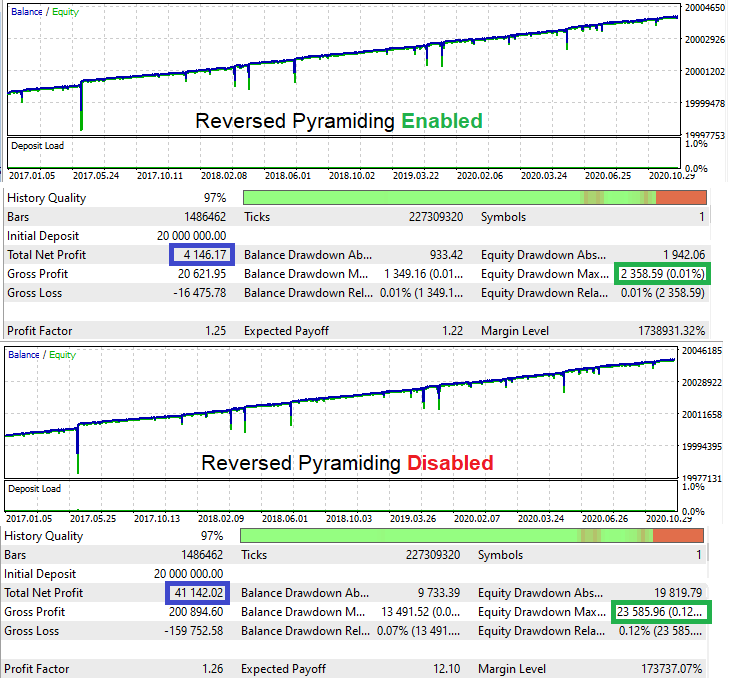## 量化课程

移动端课程